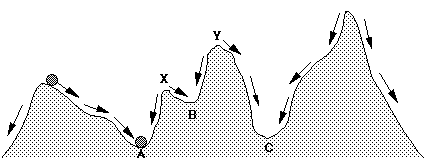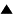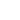# Fitness Landscapes

In many cases, the rather abstract and mathematically complex structure of a system of attractors and basins can be replaced by the more intuitive model of a fitness landscape. Under certain mathematical conditions, the deterministic dynamics of a system can be represented by a potential function F on the state space, which attaches a certain number to each state: F: S -> R: s -> F(s), such that trajectory of the system through the state space will always follow the path of steepest descent, i.e. move from a given state s to that neighboring state for which F is minimal. In mechanics, this function corresponds to the potential energy of the system. More generally, the function represents the degree to which a certain state is "preferable" to another state: the lower the value of F, the "better" or the more "fit" the state. Thus, the potential can be seen as the negative of the fitness function. It is unfortunate that the convention in physics sees systems as striving to minimize a potential function, whereas the convention in biology sees systems as striving to maximize a fitness function. Although this tends to be confusing, the two types of representation are equivalent apart from an inversion of the sign of the function.

The fitness function transforms the state space into a fitness landscape, where every point in the space has a certain "height" corresponding to its fitness value. This landscape has peaks and valleys. The attractors of the dynamics will now correspond to the local minima of the potential function (potential valleys), or, equivalently, to the maxima of the fitness function (fitness peaks). This can be understood by noting that the system will always move downward in the potential landscape. When it has reached the locally lowest point, all remaining directions will point upward, and therefore the system will not be able to leave the bottom of the valley (see figure).

The local maxima of the potential (peaks) are the points that separate the basins of the attractors (valleys) that lie in between the peaks. In general, the steeper the slope, the faster the descent of the system along that slope.Figure: a fitness landscape: the arrows denote the directions in which the system will move. The height of a position corresponds to its potential or to its lack of fitness. Thus, A has a higher fitness (or lower potential) than B. The bottoms of the valleys A, B and C are local minima of the potential, i.e. attractors. The peaks X and Y delimit the basin of the attractor B. X separates the basins of A and B, Y separates the basins of B and C.

 HomeMetasystem Transition TheoryEvolutionary TheorySelf-organization Up Prev.Next Down